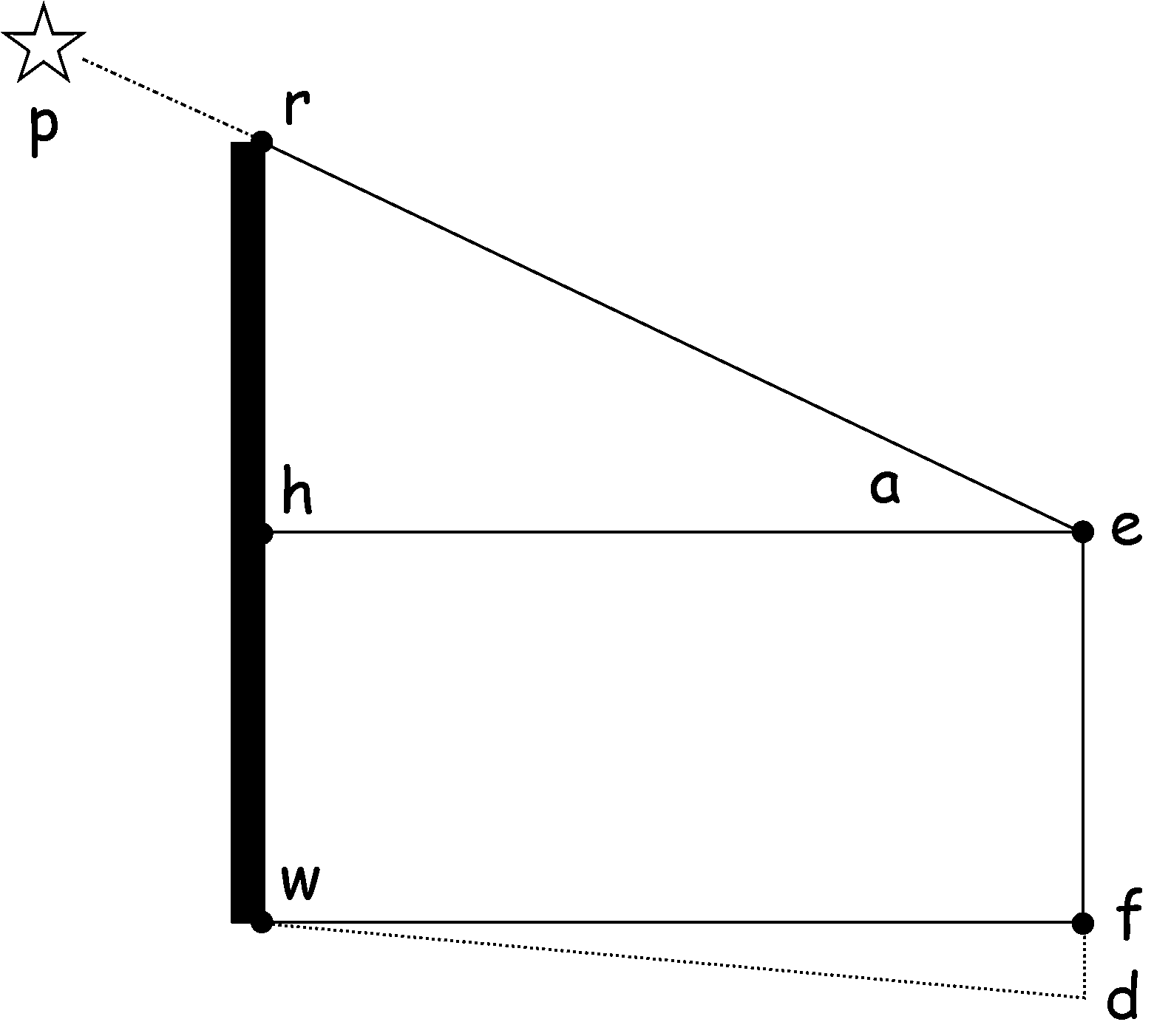# Simple Estimates of the Altitude of Polaris

In the debate about whether the earth is round of flat, certain simple observations can be made that can decide one way or the other.
One of these is measuring the altitude (angle) above the horizon of the star Polaris (North Star, Alpha Ursa Minor)
Since Polaris is almost above the North Pole it appears almost stationary whereas all other stars appear to move. This makes it a useful navigation tool.

The purpose of this page is to demonstrate how easy it is to make a basic estimate of the angle between the horizon and Polaris that anyone can do. All it takes is a tape measure and a calculator.

The author used a house in Kathmandu, Nepal to make the first measurement. All the observer has to do is stand on a level surface, line up Polaris against some point that can be measured above the ground, note the point directly beneath the observer's eye and the hight of the observer's eye above the ground.
The arrangement is shown below:Here,
p  is Polaris.
r  is some alignment point.
e  is the observer's eye (only one).
f  is the point directly beneath the observer's eye.
w  is the point directly beneath r.
h  is the horizon level with the observer's eye.
d  is a possible drop in the ground at the observer.
a  is the altitude of Polaris, what we are trying to find out.

What we need to measure is:
rw  the vertical distance from alignment point to the ground.
ef  the vertical distance between the observer's eye and the ground.
wf  the horizontal distance between the aligment point and the observer's eye.
The measurements can be simply made with a tape measure and possibly with the help of an assistant.

The following measurements were made on 11. May 2018 at approximately 20:00 Kathmandu time (14:15 GMT)
at a location of 27d 43m 32.25s (27d 43.54m; 27.7257d) North; 85d 20m 5.82s (85d 20.10m; 85.3350d) East:

rw  364cm,   ef  164cm,   rw  382cm

To calculate the angle, a, we use:

a = arctan( rh / he )
= arctan( ( rw-ef ) / wf )
= arctan( 200 / 382 )
= 27.6 degrees.

On a round earth with Polaris directly over the North Pole, the altitude of Polaris above the horizon exactly equals the observer's latitude.
Note the remarkably close agreement with the measured value and the latitude of the observing site!

Errors
Athough this result is very good, this is more likely a coincidence as there is not a high degree of acuracy in the measurements.Let's take a look at three sources of error to get an idea of the range of values we could obtain:

Measurement accuracy
Atmospheric Refraction
The light from a star does not travel in a straight line as it enters the atmosphere. It bends in such a way to make the star appear higher in the sky than it really is. This ranges from 0 degrees if the star is directly overhead to about 0.5 degrees (sun's diameter) at the horizon.
For the observation here, there are several online refraction calculators but this one[a] uses the hight of the observer. Values entered:
Observed altitude 27.7 degrees, Temperature 25 degrees, Height 1300m.
This would elevate Polaris by about 1.6 arcminutes which is only 0.027 degrees,
which does not really make any difference to this level of observation

Polaris is not directly over the North Pole
The astronimical coordinates of Polaris are: RA 2h 31m 49.09s (2h 31.8117m; 2.5302h), Dec 89d 15m 50.8s (89d 15.85m; 89.2641d)[c]
From this it can be seen that Polaris is 0.7359 degrees from the North Pole. This is almost one and a half moon diameters.
So the actual altitude at a given time can vary by 1.4718 degrees.

It is possible to calculate the actual altitude (requires round earth) and there is an online calculator avaliable.[b]
The following data was entered:

 Declination 89 degrees 15.85 minutes Right Ascension 2 hours 31.81 minutes Latitude 27 degrees 43.53 minutes Longitude 85 degrees 20.10 minutes Local Date 2018 May 11 Local Time 20:00:00

According to the calculator, the altitude of Polaris at the time of observation was 27.2 degrees and almost due north, putting it at its lowest altitude. (This does not take into acount atmospheric refraction, but as we have seen obove, this is negligable for this experiment).

13. Apr 2018